3D Geometry Primer: Chapter 2 - Issue 04 - Cartesian Equations and Normal Vectors by (01 October 2002) Return to The Archives
 IntroductionWhen I was talking to Nick about the Plane3D class that will be released in a few weeks, he mentioned the fact that all the stuff we learned last time is hardly ever used in real programs. And he was correct. Parametric equations of planes are often avoided in favor of the much smaller cartesian one. You've seen that all parametric equations of planes need at least 9 floats: e.g. 3 for the plane origin, and 2 x 3 floats for both direction vectors. As we'll see in a minute, the cartesian equation only needs 4 floats (which makes it ideal for abusing it to store RGBA colors :). Also, most operations done on planes can be done far easier by using cartesian equations. You ask, why did I even bothered about parametric equations? The cartesian equation has its limits. So you really understand what planes are. I don't know the third reason anymore. "Chocolate, of course, is the stuff of which fantasies are made. Rich, dark, velvety-smooth fantasies that envelop the senses and stif the passions. Chocolate is madness; chocolate is delight.", Judith OlneyAnyway, we'll continue with some more useless stuff: the cartesian equation for freaks...

 (I) The Cartesian Equation, The Hard WayAs for the cartesian equation for a line, we want some kind of a test that tells us if a certain point P(x, y, z) lies on a plane. What we really want is a method in which we can feed known points or known direction vectors of the plane, which results in a cartesian equation. For example, we want to be able to find the equation for the plane that goes through exactly three points. But we also want to find the equation of a plane of which we know exactly one point and two direction vectors. The way to do it is quite difficult to explain since it needs the homogeneous representation of 3D geometry (which actually uses 4D geometry). So I won't explain it in detail ...The trick is to consider our 3D plane as the intersection of two 4D hyperplanes. One represents our 3D space and consists of all points P(x, y, z, w) with w = 1. The other goes through the 4D origin O(0, 0, 0, 0) and is the "extended version" of our plane. This extension is simplified as the following: every 3D point gets an extra component value w = 1, and every vector gets an extra component value w = 0. Then we use a test that demands that four 4D points *and* the origin lie on exactly one hyperplane. This is a well know test for everybody who uses this homogeneous representation of 3D geometry, but it's beyond the scope of this chapter to explain where it comes from. The test however is very simple: you take one 4x4 matrix and fill in the four points. Then you take the determinant of that matrix and you demand it results in zero.OK, but how do we use it? You fill in four things for our 3D plane. The first is the point we want to test: P(x, y, z). Since this is a point, we extend this to 4D by adding a 1 as the w component value. You can find this back on the first line of the matrix. Second, you fill in three things you know of the plane. If it is a point, you also add a 1 as the w component value, but if it is a direction vector, we fill in a 0 as the w component value. The order of the lines doesn't matter, it won't affect the cartesian equation.I think it's best I give you some examples, isn't it :) The first I'll give you is the matrix you need if you know one point S(s1, s2, s3) and two direction vectors D(d1, d2, d3) and E(e1, e2, e3).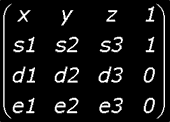The second example, exactly three points of the plane. These points are A(a1, a2, a3), B(b1, b2, b3) and C(c1, c2, c3).We'll continue now with the first example. Once you have its matrix, you take it's determinant (remark 1) and you demand that it results to 0. You will get the following equation:And if you also calculate the determinants of the 3x3 matrices (remark 2), you'll get the following equation which you all may have already encountered. And that's good, since this is the famous cartesian equation of a plane :)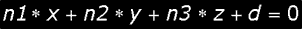Notice that the variables n1, n2, n3 and d are *new* variables, i.e. we invented these names here and they contain the results of the four determinants. You can also write this equation with the more famous names a, b, c and d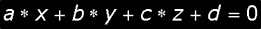Cool, and now we get smart and recognize a dot product! Put the values n1, n2 and n3 in a vector N(n1, n2, n3), and consider also the vector P = OP = (x, y, z) and you'll get the following short equation. Now you know why I used n1, n2 and n3 instead of the famous a, b and c :)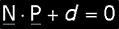You probably also recognize this equation, and yes, this vector N is called the normal vector! We'll see later why that vector is indeed the normal vector. So in short: take 4x4 matrix, fill with stuff, calculate some determinants and get the cartesian equation of the line.Notice that P is the position vector of a point P. We don't immediately use P in the equation, because you can't really calculate the dot product between a vector and a point. In fact, try it. Use the geometrical definition of the dot product which you can find in issue I.02, and try to calculate the dot product. You'll find out you can't. However, what we really want is to do the dot product between N and the postion vector of P! Hence we write P.

 (II) The Good, The Bad And The Normal VectorThere seems to be a big mystery about normal vectors of planes, but actually it's nothing special. Let's talk the talk and walk the walk...A normal vector is something that exists in every point of every smooth surface. Read that again: smoooooth surfaces. Spheres are smooth surfaces, cubes are not. Though, many many points of the cube's surface have a normal vector. The only points that don't, are the "unsmooth" points like the edges of the cube. Hence we can say: a normal vector is something that exists in every point of every smooth surface and of every smooth area of an unsmooth surface.A normal vector N is a vector that is orthogonal to a surface. Usually it is normalized and points to the outside of the surface. Imagine *you* stand on a surface (a plane or even a sphere e.g. the earth). With a bit of luck you stand orthogonal to that surface and you stand at the outside of the surface. Consider yourself to have a length of 1 meter. Then your feet are the tail of the normal vector N and your head is, well uhm, the head of N. That is the simple truth about normal vectors.Say we're on a smooth surface. In the very close neighborhood of where you stand, you'll find out that for every two points P and Q of the surface you take, if you connect them with a vector QP = P � Q er the head), then this vector QP will be orthogonal to the (local!) normal vector N. If you take the dot product between this vector QP and N you'll find out that it results to 0.This is really *really* *REALLY* important! I suggest you start all over and reading this paragraph for a second time. Then you may continue...Now you also know why there are no normal vectors for the edges of a cube. Well, try to consider a cube and a path that goes from one face to another, crossing an edge. Try to envision yourself (as a normal vector) at each point on your way on that path ,and what happens as the path crosses the edge. We want these normals to change continuously. If your path is parametric, there is some parameter t you change in order to get different points on your way. If the normals on this path are continuous, the change between two normals on your way can be made arbitrarily small by changing the dt. Like, let's say you want to make the difference in angle less than 1 degree between two "close" normals on your path. If the normals are continuous along the path, you'll will be able to choose a small enough dt such that you have to turn less than a degree to turn from normal N(t) to N(t + dt). As long as you're on one face, there's no problem since you don't have to turn at all. You can choose any dt, your rotation will be 0 degrees. However, at the edge there'll an abrupt jump in the change of normals. You always have to turn 90 degrees if you go from one face to the other. You can't make the dt small enough and thereby make the "jump" smaller, because it happens AT A SINGLE POINT as opposed to happening smoothly across an interval. This thingy about dt is called the delta-epsilon definition of continuity.

 (III) Normal Vectors For PlanesPlanes are a paradise for everyone who works with normal vectors. The reason is very simple: for each point of the plane, you will find a normal vector that points in exactly the same direction and sense and has exactly the same length as all other points. i.e.: for each point you'll find exactly the same normal vector. There is only *one* normal vector N for each plane. And that's something very unique! Nah, not quite yet. Now I'm not telling the whole truth... in general, normal vectors point to the "outerside" of the world. Many surfaces have an "outerside" and an innerside": the surface of a sphere, a cube, a cylinder, a pyramid, the surface of any body. Because all these surfaces belong to a body, you can easily determine what side of the surface points to the inside of the body, and what side points to the outside of the body. If you can do this, then you know how to point the normal vector: to the outside.Planes however don't seem to have an inner- or outerside. And believe me: they really don't have it: both sides are identical. This is because a plane is not the surface of a body. There's nothing to tell what side of the plane faces the inside. So what about it? Now you have two sides that can be the outside. That means we can have *two* normal vectors for each plane! And what's more, both normal vectors exists for each point of the plane. This isn't funny. We don't like that. For each point of a surface, we only want exactly one normal vector. Not two. So we need to eliminate one of the normal vectors. But how???The answer is very simple: you can choose one. In many situations (the context), the human logic will determine a innerside and an outerside of the plane. And by that he'll determine what normal vector to choose: Maybe a plane is used as "the floor of the world". In that case, the upperside (the front) can be seen as the outside of the floor so you'll pick the normal pointing up. Or you can see the upperside as the innerside of world, so you'll pick the normal pointing down. It depends on how you interpret the upperside of the floor: as innerside or outerside of the world.Most walls are flat, so imagine in a game a plane that is coincident with a wall. That wall is textured and has a front and back. The front is the textured side, and the back is mostly not textured and not visible. In that case, the front can be seen as the outerside of the wall, so you'll choose the normal pointing out of the wall. In most situations, you'll be able to define the front and back of a plane. If a plane is textured, you'll likely choose the textured side as the front. In general, the front is chosen as the outerside, so you'll choose the normal coming out of the front side.As you can see, it's up to you how you interpret both sides of the plane. In cases where you can't distinguish a front and a back, you can freely pick one of both normals. The math won't come up with different results if you pick the other (at least, it shouldn't ...)One last advice however: After you have chosen, it's the chosen normal vector that determines what the outside of the plane is. Stick with that! Once you have chosen your normal vector: keep using that one, keep using the same side as the outside! Don't suddenly use the other one! Big misery will happen to you! (And I know it ... :)

 (IV) The Cartesian Equation With The Super TrickHeil den Freddie. He was the person who first explained to me the following way to find the cartesian equation of a plane. Say for yourself, you don't like the method described in paragraph (I). You need to calculate 4 determinants of 3x3 matrices which envolves 4 * 6 * 2 = 48 multiplications and 4 * 5 = 20 additions. And above all, many of you don't even know about determinants. So Freddy taught us a much faster way to get the cartesian equation (9 muls and 5 adds). Let us be clear! We'll find the *same* equation! We'll only find it faster. And you know what's funny? When he showed us this trick we didn't even know about cross and dot products, while the method uses exactly one cross and one dot product! But you do know about crosses and dots, so that means that this will even be better...a) Finding the normal vector:Take two points A and B of a plane plane with cartesian equation N � P + d = 0. We can fill in both points in the cartesian equation. Then, you subtract both equations, side by side.Now we have discovered that for every two points A and B of the plane you take, you can construct the vector BA and that this vector is orthogonal to N. Hence all vectors that lie on the plane are parallel to the normal vector N. What we've done now is to prove that N is indeed the normal vector of this plane! I just stated that at the end of paragraph (I), but I didn't quite explain it. Now that we know that, we have to solve another thing...How do we find a vector that is orthogonal to all vectors AB of that plane? First, we find out that we can write any vector AB as a linear combination of both direction vectors and of the plane (if you don't believe this, take the equations for both points A and B and subtract them):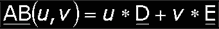Then we demand that the normal vector N is orthogonal to all these vectors AB(u, v):Because this has to be true for all possible values of u and v, this can only mean that N has to be orthogonal to both direction vectors.That this is true, we could have expected that: direction vectors are vectors of the plane too! And the normal vector N must be orthogonal to all vectors of the plane, hence also to the direction vectors.With this information, we can find candidates for the normal vector N, since you can use a cross product to find vectors that are orthogonal to two operands. Since the cross product is not commutative, we find two different candidates: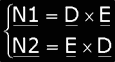This is consistent with paragraph (III). Both are valid normal vectors, so we'll have to choose one. Under normal conditions, you'll choose the first one, since that way the trio forms a right-handed trio. If you want the normal vector to point in one or the other way, you'll have to choose D and E in a corresponding way.Remark: One more thing, which is quite important: You see that for N I divide by the norm of the cross product. This is because nor N1 and N2 will be normalized, even if D and E *ARE* normalized! This is because the angle between D and E determine the length of the cross product too. Hence you'll have to normalize it yourself!b) Finding dThis is really simple: put the just found normal vector N and one known point of your plane into the cartesian equation and solve for d. As the known point, we'll use the support point S but any point of the plane can do:c) In short...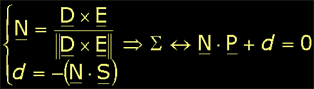d) Alternative ...The cartesian equation we have used thus far for planes is the one that you'll mostly use in graphics. Mathematicians often use another one without the d value. They do this by keeping the support point S around, and simply substitute the equation for d in the cartesian equation. They end up with this: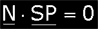Verify that this is correct. The reason why they prefer this one is because it only uses two vectors and one dot operation. This allows them to use vector arithmetic easier. Maybe you'll find yourself in a situation where you'll more like to use this equation. Anyway, now you have two possible ones, but the first one is mostly more efficient.That's it for today. Next time, we'll talk even more on planes. Then we'll also release the plane classes.With grateful thanks to Per Vognsen to help my tired brain out of this silly mess,See you later alligators! Bramzilla

 (1) The determinant of a 4x4 matrix is calculated by using determinants of 3x3 matrices:(2) The determinant of a 3x3 matrix is calculated as next: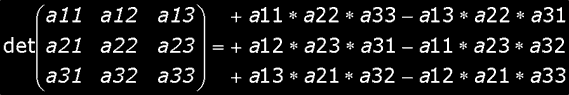Article Series:3D Geometry Primer: Chapter 2 - Issue 01 - Appendix3D Geometry Primer: Chapter 2 - Issue 01 - Points And Lines3D Geometry Primer: Chapter 2 - Issue 02 - Appendix3D Geometry Primer: Chapter 2 - Issue 02 - More About Lines3D Geometry Primer: Chapter 2 - Issue 03 - Planes and Parametric Equations3D Geometry Primer: Chapter 2 - Issue 04 - Cartesian Equations and Normal Vectors